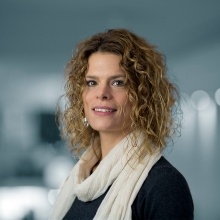# Computational Methods for Uncertainty Quantification

Research Group

Bridging the gap between stochastic analysis and numerical simulations.

Mathematical models to describe natural phenomena are often based on partial differential equations. The inputs to the equation, such as coefficients, boundary or initial conditions, the data or the domain itself may not be known a-priori. Insufficient or imprecise measurements have to be accounted for when the model is supposed to be realistic. The equation itself could, further, be chaotic. Stochastic partial differential equations account for all sorts of uncertainties and chaotic behaviour. The aim of the group is to investigate solution concepts for stochastic partial differential equations, analyse solutions and invent numerical methods to approximate solutions, hence, bridge the gap between stochastic analysis and numerical simulations. The methods developed have applications in various natural and engineering sciences.

## News & Events

### ContactProf. Dr.